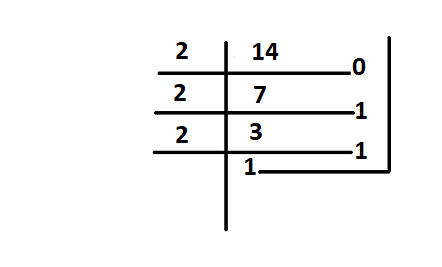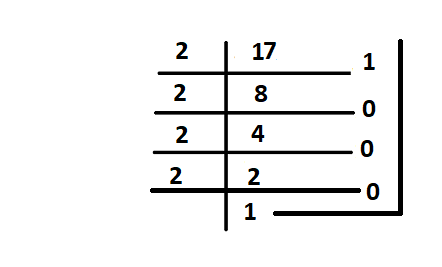GeeksforGeeks App
Open AppBrowser
Continue

# How many types of number systems are there?

The number system includes different types of numbers for example prime numbers, odd numbers, even numbers, rational numbers, whole numbers, etc. These numbers can be expressed in the form of figures as well as words accordingly. For example, the numbers like 40 and 65 expressed in the form of figures can also be written as forty and sixty-five.

A Number system or numeral system is defined as an elementary system to express numbers and figures. It is the unique way of representing of numbers in arithmetic and algebraic structure.

Numbers are used in various arithmetic values applicable to carry out various arithmetic operations like addition, subtraction, multiplication, etc which are applicable in daily lives for the purpose of calculation. The value of a number is determined by the digit, its place value in the number, and the base of the number system. Numbers generally are also known as numerals are the mathematical values used for counting, measurements, labeling, and measuring fundamental quantities.

Numbers are the mathematical values or figures used for the purpose of measuring or calculating quantities. It is represented by numerals as 2, 4, 7, etc. Some examples of numbers are integers, whole numbers, natural numbers, rational and irrational numbers, etc.

### Types Of Numbers

There are different types of numbers categorized into sets by the number system. The types are described below,

• Natural numbers: Natural numbers are the positive numbers that count from 1 to infinity. The set of natural numbers is represented by ‘N’. It is the numbers we generally use for counting. The set of natural numbers can be represented as N = 1, 2, 3, 4, 5, 6, 7,…
• Whole numbers: Whole numbers are positive numbers including zero, which counts from 0 to infinity. Whole numbers do not include fractions or decimals. The set of whole numbers is represented by ‘W’. Example: W = 0, 1, 2, 3, 4, 5,…
• Integers: Integers are the set of numbers including all the positive counting numbers, zero as well as all negative counting numbers which count from negative infinity to positive infinity. The set doesn’t include fractions and decimals. The set of integers is denoted by ‘Z’. Example: Z = …..,-5, -4, -3, -2, -1, 0, 1, 2, 3, 4, 5,…
• Decimal numbers: Any numeral value that consists of a decimal point is a decimal number. It can be expressed as 2.5, 0.567, etc.
• Real number: Real numbers are the set numbers that do not include any imaginary value. It includes all the positive integers, negative integers, fractions, and decimal values. It is generally denoted by ‘R’.
• Complex number: Complex numbers are a set of numbers that include imaginary numbers. It can be expressed as a+bi where “a” and “b” are real numbers. It is denoted by ‘C’.
• Rational numbers: Rational numbers are the numbers that can be expressed as the ratio of two integers. It includes all the integers and can be expressed in terms of fractions or decimals. It is denoted by ‘Q’.
• Irrational numbers: Irrational numbers are numbers that cannot be expressed in fractions or ratios of integers. It can be written in decimals and have endless non-repeating digits after the decimal point. It is denoted by ‘P’.

### What is a number system?

A Number system is a method of showing numbers by writing, which is a mathematical way of representing the numbers of a given set, by using the numbers or symbols in a mathematical manner.

The writing system for denoting numbers using digits or symbols in a logical manner is defined as a Number system. The numeral system Represents a useful set of numbers, reflects the arithmetic and algebraic structure of a number, and Provides standard representation. The digits from 0 to 9 can be used to form all the numbers.

With these digits, anyone can create infinite numbers. For example, 156,3907, 3456, 1298, 784859 etc.

### Types of Number Systems

Based on the base value and the number of allowed digits, number systems are of many types. The four common types of Number systems are:

• Decimal Number System
• Binary Number System
• Octal Number System

Decimal Number System

Number system with a base value of 10 is termed a Decimal number system. It uses 10 digits i.e. 0-9 for the creation of numbers. Here, each digit in the number is at a specific place with place value a product of different powers of 10. Here, the place value is termed from right to left as first place value called units, second to the left as Tens, so on Hundreds, Thousands, etc. Here, units have the place value as 100, tens have the place value as 101, hundreds as 102, thousands as 103, and so on.

For example, 12265 has place values as,

(1 × 104) + (2 × 103) + (2 × 102) + (6 × 101) + (5 × 100)

= (1 × 10000) + (2 × 1000) + (2 × 100) + (6 × 10) + (5 × 1)

= 10000 + 2000 + 200 + 60 + 5

= 12265

Binary Number System

Number System with base value 2 is termed as Binary number system. It uses 2 digits i.e. 0 and 1 for the creation of numbers. The numbers formed using these two digits are termed Binary Numbers. The binary number system is very useful in electronic devices and computer systems because it can be easily performed using just two states ON and OFF i.e. 0 and 1.

Decimal Numbers 0-9 are represented in binary as: 0, 1, 10, 11, 100, 101, 110, 111, 1000, and 1001

For example, 14 can be written as 1110, 19 can be written as 10011, 50 can be written as 110010.

Example of 14 in the binary systembinary 14

Here 14 can be written as 1110

Octal Number System

Octal Number System is one in which the base value is 8. It uses 8 digits i.e. 0-7 for the creation of Octal Numbers. Octal Numbers can be converted to Decimal values by multiplying each digit with the place value and then adding the result. Here the place values are 80, 81, and 82. Octal Numbers are useful for the representation of UTF8 Numbers. Example,

(81)10 can be written as (121)8

(125)10 can be written as (175)8

Number System with base value 16 is termed as Hexadecimal Number System. It uses 16 digits for the creation of its numbers. Digits from 0-9 are taken like the digits in the decimal number system but the digits from 10-15 are represented as A-F i.e. 10 is represented as A, 11 as B, 12 as C, 13 as D, 14 as E, and 15 as F. Hexadecimal Numbers are useful for handling memory address locations. Examples,

(185)10  can be written as (B9)16

(5440)10  can be written as (1540)16

(4265)10  can be written as (10A9)16

### Sample Questions

Question 1: Convert 45258 into a decimal?

Solution:

45258 = 4 × 83 + 5 × 82 + 2 × 81 + 5 × 80

= 4 × 512 + 5 × 64 + 2 × 8 + 5 × 1

= 2048 + 320 + 16 + 5

= 238910

Question 2: Convert (17)10 as a binary number?

Solution:binary 17

Therefore (17)10 = (10001)2

Question 3: Convert (1011110)2 into an octal number.

Solution:

Given (1011110)2 a binary number, to convert it into octal number

Using this table we can write give number as

001 011 110 i .e

001 = 1

011 = 3

110 = 6

So (1011110)2 in octal number is (136)8

My Personal Notes arrow_drop_up
Related Tutorials